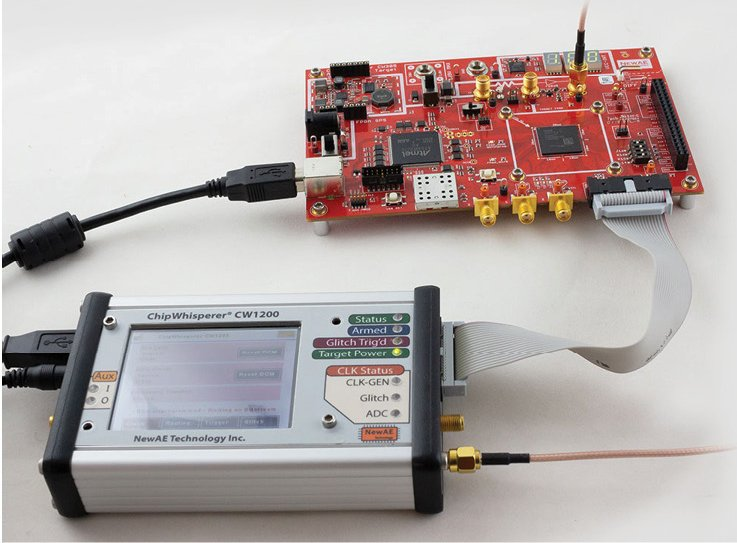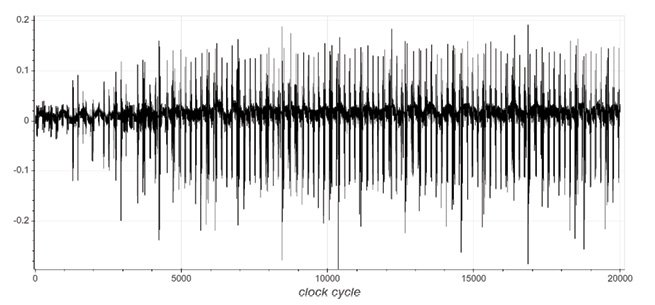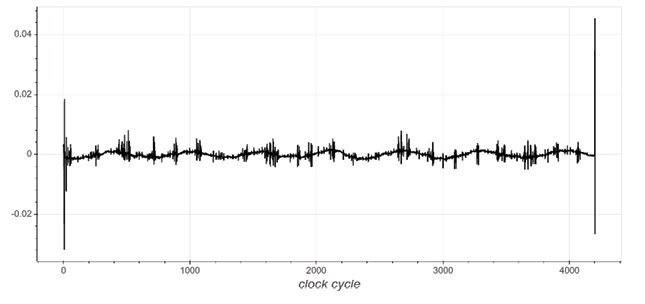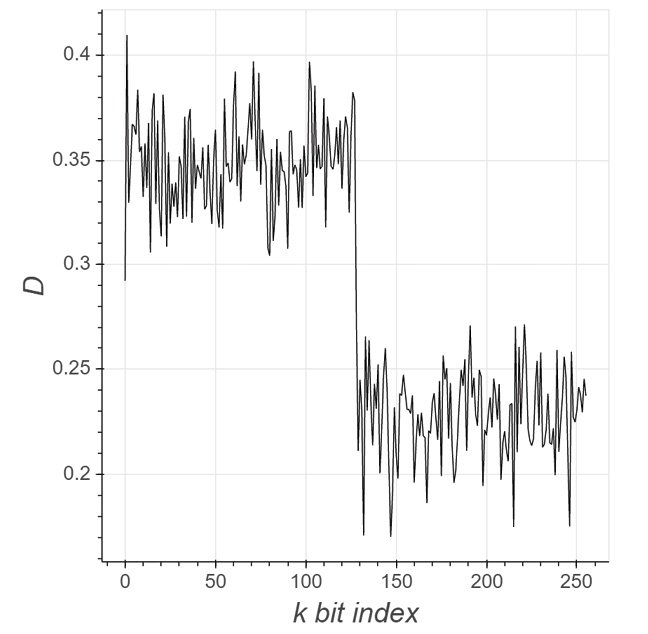# Power Analysis of ECC Hardware ImplementationsWritten by

## Curves and Keys

### Power analysis can recover cryptographic keys from implemented algorithms. In this article, Colin works through such an attack on an ECC hardware implementation, showing how you can identify leakage of key material that makes an attack possible.

• How power analysis can recover cryptographic keys from implemented algorithms.

• How attestation works

• How to understand the point multiply operation in ECC

• What are the steps in power analysis?

• How to plot the actual results

• How to understand the hidden number problem (HNP)

• NewAE Technology CW305 target board

• ECDSA (elliptic curve digital signature algorithm)

• Power analysis

This month I’m going to take you through a side channel attack on a hardware elliptic curve cryptography (ECC) implementation. This might be new to you, as I’ve found that ECC isn’t as well-known or used as AES (advanced encryption standard). I’ve covered attacks on AES in many previous issues—including fault attacks, and power analysis on software and hardware implementations of AES.

I won’t cover a full introduction to ECC, because it turns out that even attacking it doesn’t require you to know all the details. But, if you’re looking for a friendly introduction, my favorite reference is the book “Serious Cryptography” by Jean-Philippe Aumasson. The important difference compared to algorithms such as AES is that ECC is an asymmetric algorithm.

With asymmetric cryptography we have a public and private key pair. This is very powerful as it means we can use ECC for more than just encrypting data. We can use it as part of a signature operation, where the freely available public key can be used to verify a given signature is correct. This also allows us to use it for attestation, an important operation in many cloud operations.

##### PUBLIC KEY

With attestation, our end device has a private key securely stored inside it. When the device is first registered to the cloud server, the cloud server remembers the device’s public key. This public key isn’t a secret, so there is no risk of it being leaked by the cloud server. But only the device with the private key associated to that public key can correctly sign messages that will verify with the given public key. Thus, the cloud server can forever confirm a connected device is the one that originally provided a given public key.

This means that the secret private key never needs to leave the device. A well-designed system wouldn’t even have a way of reading out the private key from the device, since it would never be needed. The device only ever needs to sign messages. In theory this is a very secure setup, since the cloud server can be thoroughly hacked, as it only ever stores the public keys for the device.

The manufacturer can therefore always trust a given device is authentic, since only devices with known public keys that the manufacturer initially registered are trusted as authentic. The only way to fake this would be to read the private key from an end device—something that’s impossible by design. Of course, you know in my columns I like showing you the way around impossible. So that is exactly what we’re going to do. We’ll see how you can recover the private ECC key used in a P-256 ECDSA engine. ECDSA stands for “elliptic curve digital signature algorithm.”

This article includes substantial contributions by several colleagues who I want to acknowledge here. Jean-Pierre Thiabault built up this ECC attack I’m discussing, and Alex Dewar helped improve some of the tooling that I’m using. In addition, the ECC core I’m going to be using is from the CrypTech Project, and was written by Pavel Shatov.

##### POINT MULTIPLY

When it comes to ECC, the important operation we consider is called the point multiply. This operation is typically referred to as kP, where k is a scalar value and P is the point on the elliptic curve. There are normally different curves that will be in use, and the fundamental idea and algorithm is the same, there are variations for the actual curves. A common curve is referred to as P-256, which references to one of several curves specified by the US NIST organization.

Fundamentally, if we can recover the value of k used for a signing operation, we can recover the private key and thus break the security assumption. Typically, we can safely assume that we can either force the device to perform such signing operations or simply monitor it during legitimate usage.

In order to explore this more, we need an ECC implementation to analyze. Because the objective of this article is to give you a way to learn about ECC attacks, I’m going to use an open-source ECC core. In particular, I’m using cores from the CrypTech project. This project started in 2015 and has developed several open-source cryptographic cores. The objective of CrypTech was to build “trustable” cryptography we can use to underpin a completely trusted chain of devices.

These cores are not designed to be side-channel resistant. That’s because they are designed to be used securely locked away from physical access. But they are one of few true open-source hardware implementations, so they give us a better example of a core to look at to understand the leakage and operation. The actual leakage we see on this core will be similar to what we’ll see on real hardware devices.

##### POWER ANALYSIS FIRST STEPS

I’m using my NewAE Technology CW305 target board, shown in Figure 1. This has the advantage of being “purpose built” for performing the types of power analysis attacks I’m going to demonstrate. Of course, if this was a real device, we’d first need to instrument the device to measure the power consumption from it.FIGURE 1 – CW305 FPGA target board with Artix A35 or A100 device

Onto this device we load the ECDSA core from CrypTech. We can now request that the core perform signing operations, and take a first look at a power trace. An overview of the power trace is given in Figure 2, which shows the core performing a point multiply operation (which is the core of the signing operation).FIGURE 2 – An overview of a full point-multiply operation, viewed as a power measurement

We can measure the time it takes to confirm the implementation is constant time, in this case we find it takes 1,124,157 clock cycles. This cycle count doesn’t change with different values of k or P (the inputs to the point multiply), which is a good sign. If the execution time depended on the inputs, this would be a bad sign that there is a serious issue with the implementation leaking secret information!

As a next step, we might explore the difference when the core is processing a zero bit versus a one bit in the secret key. A very basic implementation will have obvious differences in the timing between a zero and one bit. We can see a view of the power during processing of four bits of key in Figure 3. Each bit takes exactly 4,204 cycles to process. Several windows of zero and one bits have been overlaid using different colors. You might notice small variations, but there is no obvious major difference between the power traces, again this suggests that this is a well-designed implementation without very obvious issues.FIGURE 3 – Overlaying the processing of several bits shows little visually obvious difference between a one and zero being processed

From here we move onto the next stage of our power analysis attack: performing a differential power analysis attack by subtracting a power trace with a one bit and a power trace with a zero bit. While the shapes might be the same, perhaps there is more obvious information hidden in the actual power used.

##### SPLITTING THE DIFFERENCE

Differential power analysis is a topic I’ve covered several times before on both software and hardware implementations of AES. A good overview of them was in my article “Side-Channel Power Analysis” (Circuit Cellar 314, March 2019) , which showed where these power differences come from when processing a zero versus a one bit.

So, if we do assume there is then some difference when a zero and a one is processed, we need to evaluate the traces to confirm this. The “easy” way to do this would be on a device we fully control. In this case we cause it to execute a number of operations with a series of random (but known) secret bits. We can take a single power trace and extract the portions of the power traces where we know a one is being processed, and compare it to when a zero is being processed.

With any luck, we’ll see some areas of the trace that have a noticeable difference. This is shown in Figure 4. The major spikes are right at the start and end of each bit, in this figure specifically around clock cycles 6, 7, 4202 and 4203. You’ll notice there is a large (narrow) spike at the start and end. This suggests that we can actually make a “distinguisher” by adding up those specific samples for a given bit. This distinguisher will tell us if a given bit is a zero or one.FIGURE 4 – Subtracting the one and zero processing shows more obvious spikes at the start and end of the processing cycle

The specific spike has both positive and negative portions, so we’ll sum the samples together with a -1 or +1 scaling factor to create a positive result. The example distinguisher in this case would be:

Since the point multiplication is constant time, we know exactly when each bit is processed. This allows us to plot for example the value of the distinguisher for bit 0, which we call D0. As there are 256 bits, we thus have D0, D1, …, D255 being the output of this distinguisher equation for each bit being processed.

To validate our distinguisher seems to make sense, we can plot the output of this distinguisher for each bit with the secret k value set to a known value. To make this obvious, the secret k is set with the first half as 1s and the second half as 0s. The result looks as in Figure 5, which is an average of 5 traces.FIGURE 5 – Using identified “leaky” samples, we plot the distinguisher over a known secret k value

The Y-axis (D) is the value of the distinguisher equation. You’ll notice that there is a defined interval when we could make a decision if that bit is zero or one, and it would correctly identify all of the one and zero bits.

##### PLOTTING ACTUAL VALUE

Another question that will then come up: of those four sample locations (6, 7, 4202, 4203) did any specifically contribute more or less to the distinguisher? We can answer this by plotting the actual value of those sample numbers, as shown in Figure 6. This uses a different bit pattern than previously, and is averaged over more traces than in Figure 5. In this case 50 traces are used in the average, compared to the 5 used in Figure 5. But you can see that we can still detect single bits of one or alternating one and zero sequences.FIGURE 6 – Using a more complex secret k, we can see the specific contribution of each leaky sample

The specific bit pattern used in Figure 6 is represent with hex value:

0000ffffffffff0000000000
00ffff00aaaa0000cccc00
001111000033330000

… which contains several different types of sequences. This includes alternating two and one-bit patterns for example. This allows us to evaluate whether we are seeing an overall changing average, or we have detailed enough information to specifically pick out single bits from the power trace.

At this point, you should be able to see that these power traces can very clearly leak information about the secret bits. But I’ve also cheated a bit, because I’ve averaged several power traces. In real life we can’t average several traces, as the value of k will change each time that ECDSA is called. The value k isn’t directly “private key” like it would be with AES, but knowledge of k is sufficient to recover the true private key. This requires one additional step for a final attack, where we have a varying k, partial knowledge of the k used each time via a side channel power analysis, and we want to recover the true private key.

##### A HIDDEN NUMBER

The final step you’ll need to apply for a real attack is using the hidden number problem (HNP). While I won’t detail the complete attack here, I want to point out that the work done to demonstrate the power analysis leakage is highly practical against the typical ECDSA use-case.

With the hidden number problem, we attempt to recover the secret value with imperfect information about the leakage. A more useful version of this is used in practice, called the Extended Hidden Number Problem (EHNP). Such an attack was used in the work “A Side Journey to Titan” by Victor Lomne and Thomas Roche .

This means we don’t need to artificially constrain our signing operation for the attack to work. Instead, we can observe several (tens to thousands) of signing operations, and from those we recover enough information for the attack to succeed. In practice this is fairly straightforward, since for most devices they will allow the “safe” signing operation to be triggered by an external actor.

If you’d like to try this attack yourself, we’ve published the complete Python scripts as part of the ChipWhisperer project’s online Jupyter tutorials. The GitHub link is at . While the attack is designed to “just work” with my NewAE Technology ChipWhisperer capture hardware and a CW305 board, everything is open-source. This means you can also run this on another FPGA target board if you wish, and use any oscilloscope on your bench to perform the power measurements.

Hopefully this gives you a good starting point to evaluate how ECC performs in power analysis attack scenarios. If you are working on an IoT device that deals with attestation, thinking about how an attacker could recover the ECC private key is important to consider. If you know it’s possible for an attacker to recover the private key, you’re more likely to spend time making sure your system detects possible cloned devices. And so, I think this knowledge is the first step to designing truly secure systems that deal with ECDSA.RESOURCES

References:
 “Side-Channel Power Analysis” (Circuit Cellar 314, March 2019)
 “A Side Journey to Titan” by Victor Lomne and Thomas Roche — available at https://ninjalab.io/wp-content/uploads/2021/01/a_side_journey_to_titan.pdf
 The GitHub link as at www.github.com/newaetech/chipwhisperer-jupyter
NewAE Technology | www.newae.com

PUBLISHED IN CIRCUIT CELLAR MAGAZINE • JULY 2021 #372 – Get a PDF of the issue

 Keep up-to-date with our FREE Weekly Newsletter! Don't miss out on upcoming issues of Circuit Cellar. Subscribe to Circuit Cellar Magazine Note: We’ve made the May 2020 issue of Circuit Cellar available as a free sample issue. In it, you’ll find a rich variety of the kinds of articles and information that exemplify a typical issue of the current magazine. Would you like to write for Circuit Cellar? We are always accepting articles/posts from the technical community. Get in touch with us and let's discuss your ideas.